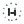0%
0%

# Arduino Capacitance Meter

We have a cost-effective capacitance meter to measure capacitance using Arduino.

Similar projects worth following
207 views
In this project, we have described how the capacitance meter will measure capacitance using Arduino.

About Project

555 Timer IC

The output signal frequency relies on R1, R2 resistors, and capacitor C1. The equation is given as,

Frequency (F) = 1/ (Time period) = 1.44/ ((R1+R2*2)*C1).

By placing the resistance and capacitance values in the equation we will see the frequency of output square wave.

Frequency (F) = 1/ (Time period) = 1.44/ (21000*C).

From the above formula, we can find out the capacitance

Capacitance C = 1.44/ (21000*F)

The Uno has a specific function pulseInwhich allows us to ascertain the positive state duration or negative state duration of a precise rectangular wave.

After connecting the unknown capacitor to the 555 timer circuit which generates a square wave output and whose frequency is instantly associated with the capacitance of the capacitor.

This signal is provided to UNO through the Schmitt Trigger gate. The UNO estimates the frequency. Using this frequency we program the UNO to estimate the capacitance with the above formula.

• 1 × Arduino UNO & Genuino UNO
• 1 × 555 timer IC
• 1 × IC 74HC14 Schmitt trigger gate
• 2 × Resistor 1k ohm
• 1 × Resistor 10k ohm
• 1
Run a Program

#include

LiquidCrystal lcd(2, 3, 4, 5, 6, 7);

int32_t Htime;
int32_t Ltime;
float Ttime;
float frequency;
float capacitance;

void setup()
{
pinMode(8,INPUT); //pin 8 as signal input
lcd.begin(16, 2);
lcd.setCursor(0,0);
lcd.print("capacitance =");
}
void loop()
{
for (int i=0;i

Share

## Similar Projects

Project Owner Contributor

### Inductance LC Meter Using ArduinohIOTron

# Does this project spark your interest?

Become a member to follow this project and never miss any updates